Courses

# MCQ : Areas Related To Circles - 2

## 10 Questions MCQ Test Mathematics (Maths) Class 10 | MCQ : Areas Related To Circles - 2

Description
This mock test of MCQ : Areas Related To Circles - 2 for Class 10 helps you for every Class 10 entrance exam. This contains 10 Multiple Choice Questions for Class 10 MCQ : Areas Related To Circles - 2 (mcq) to study with solutions a complete question bank. The solved questions answers in this MCQ : Areas Related To Circles - 2 quiz give you a good mix of easy questions and tough questions. Class 10 students definitely take this MCQ : Areas Related To Circles - 2 exercise for a better result in the exam. You can find other MCQ : Areas Related To Circles - 2 extra questions, long questions & short questions for Class 10 on EduRev as well by searching above.
QUESTION: 1

Solution:
QUESTION: 2

### Area of the largest triangle that can be inscribed in a semi-circle of radius r units is

Solution: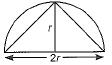Area of triangle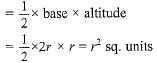QUESTION: 3

### The ratio of area of two circles whose ratio of circumference is 3:1 will be

Solution:
QUESTION: 4

Match the columns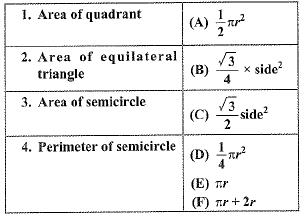Solution:

Formulae

QUESTION: 5

It is proposed to build a single circular park equal in area to the sum of areas of two circular parks of diameters 16 m and 12 m in a locality. The radius of the new park would be

Solution:
QUESTION: 6

ABC is an equilateral triangle. The area of the shaded region if the radius of each of the circle is 1 cm, is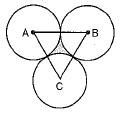Solution:

Side of triangle = 2 cm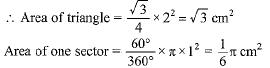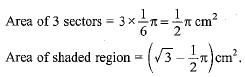QUESTION: 7

The area of the square that can be inscribed in a circle of radius 8 cm is

Solution:
QUESTION: 8

ABCDEF is any hexagon with different vertices A, B, C, D, E and F as the centres of circles with same radius r are drawn. The area of the shaded portion is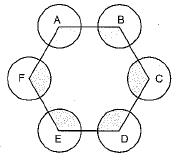Solution:

Internal angles of a regular Hexagon = 120°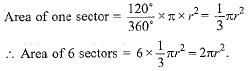QUESTION: 9

The diameter of a circle whose area is equal to the sum of the areas of the two circles of radii 24 cm and 7 cm is

Solution:
QUESTION: 10

The diameter of a wheel is 1 m. The number of revolutions it will make to travel a distance of 22 km will be

Solution: